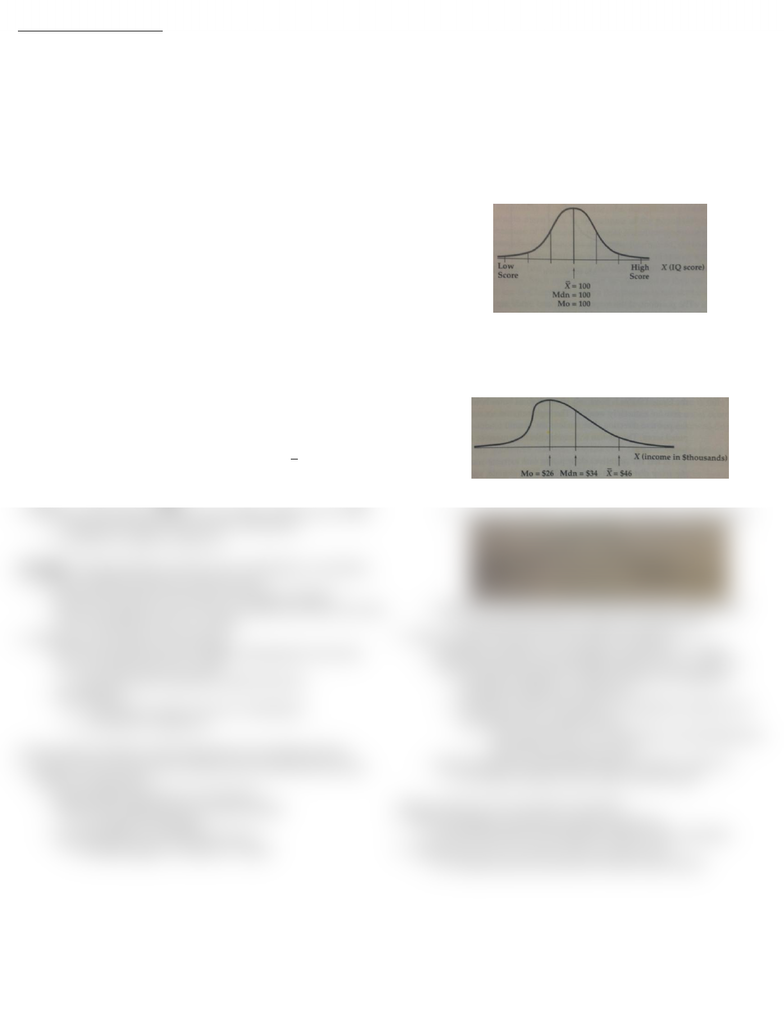# SOC202H1 Chapter Notes -Frequency Distribution, Sample Size Determination, Central Tendency

8 views1 pages
School
Department
CourseCH4 MEASURING AVERAGES
CENTRAL TENDENCY STATISTIC (CTS) provides an estimate of the typical,
usual or normal score found in a distribution of raw scores
o Three central tendency statistics, each has strengths/weaknesses
THE MEAN The sum of all scores in a distribution divided by the number of
scores observed (sample size)
Most useful of central tendency statistic for summary of typical/avrg score
o Conceptually: mean = value of the scores in the distribution if every
subject has the same score “equal share”
Applicable to interval/ratio variables only
To combine 2 different size samples
o Add sum of all scores of both groups divided by total sample size
Weakness: easily affected by extremes (lows/highs) Outliers
o 1. Sensitive to values of the scores in a distribution
Can positively or negatively inflate sum of all scores
o 2. Sensitive to sample size
Outliers especially problematic w/ smaller samples
o Adjusted Mean mean calculated w/ outliers removed
THE MEDIAN The middle score in a ranked distribution
Value which divides the distribution of scores in half
o Half the case falls above, half the case falls below
Median is a location point, the middle position score
o Useful for when distribution is skewed, not as susceptible to outliers
Different from midrange = halfway point btwn min. & max. values of X
To calculate, first rank; then divided n by 2 & get near the middle score
o If n is odd, median will be an actual sample
o If n is even, median is average btwn the two middle scores
o Larger sample:
 
 
Then find the value at that rank location
Applicable to interval/ratio variables b/c need calculate difference
Weaknesses: 2 samples w/ different mean values can have same median
o 1. Insensitive to values of the scores in a distribution
o 2. Sensitive to change in sample size
THE MODE Most frequently occurring score in a distribution, not majority
Useful w/ variables of all levels of measurements
o Mode easily spotted in charts (pie, bar, histogram, polygon)
o Special case: when all scores of X are essentially same value, the mode
is less misleading than mean or median
Weaknesses: least useful central tendency
o Narrow informational scope suggest nothing about scores that
occur around the mode score value
Only useful when reported w/ median and mean
1. Insensitive to values of scores in a distribution
2. Insensitive to Sample size
Central Tendency Statistics and the Appropriate Level of Measurement
Variable’s level of measurement indicates what mathematical formulas,
statistics are appropriate
o Mean/median appropriate w/ interval/ratio
o Mean/median inappropriate w/ ordinal variables
Ex. 0.5 gender meaningless
o Mode applicable to all measurement levels
Ex. Modal religion is “Christian” or “Islam”
FREQUENCY DISTRIBUTION CURVES A substitute for frequency histogram or
polygon in which we replace these graphs with a smooth curve.
AUC represents the total number of subjects in the population
o Equal to a proportion of 1.00 or percentage of 100 percent.
Smooth curve == estimate way scores are distributed in the population
o Not depict sample distribution
Horizontal axis = scores of a variable X; vertical axis = p/% frequency
NORMAL DISTRIBUTION a frequency distribution curve in which the
mean, median and mode of a variable are equal
o Distribution itself has bell shaped
o Median splits a ranked distribution of scores in half, symmetrical
o Mode = center point = peak of distribution
SKEWED DISTRIBUTION a frequency distribution curve in which the mean
median, and mode of the variable are unequal and many of the subjects
have extremely high/low scores
o Positively Skewed Distribution (Right Skew) extreme scores in the
high or positive end of the score distribution
High extreme inflates mean, mode unaffected, median inbtwn
o Negatively Skewed Distribution (Left Skew) extreme scores in the
low or negative end of the score distribution
Low extreme deflates mean, mode unaffected, median inbtwn
o If median isn’t btwn mean and mode distribution oddly shaped
Ex. bimodal distributions (2 peaks) for men & women
Skew in sample data doesn’t imply skewed in population
o Possibly b/c sampling error; possibly corrected by a 2nd sample
o When distribution not skewed/oddly shaped mean = ideal CTS
b/c greater flexibility vs. median & mode w/ no additional
worthwhile mathematical operations
if skewness statistic’s abs value > 1.6 regardless sample size
the distribution is probably skewed
Then sample mean != est. population central tendency b/c
distortion from extreme scores
o When distribution skewed/oddly shaped median = ideal CTS
b/c median minimizes since its btwn mean & mode
Mixing Subgroups in the Calculation of the Mean
Mean susceptible to distortion by outliers & extremes
o Req. describe which cases/subject included in mean calculation
Mixing status ranks results: mean fits no group at all
o Ex. Company salary mix executives w/ blue-collar workers
Unlock document

This preview shows half of the first page of the document.
Unlock all 1 pages and 3 million more documents.

# Get access

\$10 USD/m
Billed \$120 USD annually
Homework Help
Class Notes
Textbook Notes